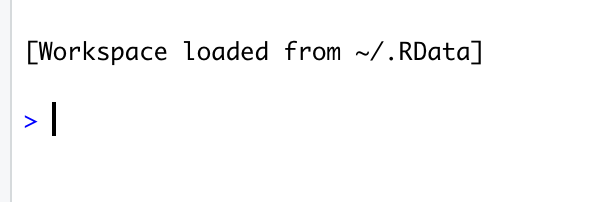# 【R语言入门】R语言中的变量与基本数据类型

## 说明

1. `R` 语言有哪些基本操作
2. 什么是变量，以及如何给变量赋值
3. `R` 语言有哪些基本数据类型，如何确定变量的数据类型

## R 语言的基本操作

`R` 语言的默认提示符是 `>` ，它表示正在等待输入命令，每次输入命令后敲击回车即可执行当前命令。`R` 语言支持的基本操作主要有加、减、乘、除、取余和幂运算，对应的运算符分别为：+、-、*、/、%%、^。

``````> 9/2
 4.5
> 9/4
 2.25
> 11/3
 3.666667``````

``````> 9 %% 2
 1
> 9 %% 4
 1
> 9 %% 3
 0
> 11 % 4

``````> 2 ^ 3
 8
> 3 ^ 2
 9
> 2 ^ 10
 1024
> 2 ^ 100
 1.267651e+30``````

## R 语言中的变量

`R` 语言中，可以将变量想象成一个盒子，我们可以将任何数据暂存到这个盒子里，但同一时刻，这个盒子只能保存一个数据，如果被多次赋值，则只会保存最后一次放入的数据。

`R` 语言中的赋值符号是 `->`，虽然也可以使用 `=` 进行赋值，但强烈建议不要这样做，这样不仅显得不专业，而且在一些情况下会出现问题。

``````> x <- 3
> x <- 4
> x <- 9
> x
 9``````

`R` 语言中的变量不需要事先声明，直接使用即可。如果使用了一个之前没有出现过的变量，则会创建一个新的变量。`R` 语言中变量是区分大小写的，`x``X` 是两个不同的变量。

``````> X

> X <- 1
> X <- 3
> X
 3
> x
 9``````

1. 只能使用字母（区分大小写）、数字、“_”（下划线）、“.”（英文句号），不能有中文、空格和其它特殊字符存在
2. 不能以数字、下划线开头，开头必须是英文字母或者点
3. 可以以点号开头，但点号后面的符号不能是数字

`R` 语言中，如果非要搞一些骚操作，用一些奇怪的变量名，也不是不可以，需要用反引号来包裹。

``````> `* 90` <- 10
> `* 90`
 10
> `变量` <- 20
> `变量`
 20``````

## R 语言中的基本数据类型

`R` 语言中主要数据类型有：向量、列表、矩阵、因子、数据框、数组。这些会在后面的文章中进行介绍，本篇只介绍基本的几种数据类型。

### 1. 逻辑型(logical)

``````> v <- TRUE
> class(v)
 "logical"
> y <- FALSE
> class(v)
 "logical"
> y <- false

`class` 函数可以获取变量的类型信息，可以看到，变量 `v``y` 都是 `logical` 类型。需要注意的是 `TRUE``FALSE` 必须全部大写，否则会出现上面的错误提示。

### 2. 数值型(numeric)

`R` 语言中数字的默认类型为数值型，在运算时需要注意其精度问题，当整数部分大于等于7位时，将舍弃小数部分，当整数部分小于7位时，与小数部分一起最多保留7位数字。

``````> i <- 10
> class(i)
 "numeric"
> j <- 11.1
> class(j)
 "numeric"
> k <- 1.1234567890
> class(k)
 "numeric"
> k
 1.123457
> k <- 123456789.123456789
> k
 123456789
> k <- 1234567.123456789
> k
 1234567
> k <- 123456.123456789
> k
 123456.1``````

### 3. 整数型(integer)

`R` 语言中，整数型需要使用数字+L来表示，如：`2L``11L``-10L`

``````> i <- 1L
> class(i)
 "integer"
> j <- 22L
> class(j)
 "integer"
> k <- -10L
> class(k)
 "integer"``````

### 4. 字符型(character)

`R` 语言中字符型即字符串类型，通常为用单引号或双引号包围的字符串。

``````> name <- "Frank"
> class(name)
 "character"
> doc_name <- "study.mp4"
> class(doc_name)
 "character"``````

### 5. 复数型(Complex)

`R` 语言是支持复数计算的，复数也是 `R` 语言的基本类型之一。

``````> c1 <- 3 + 2i
> class(c1)
 "complex"
> c2 <-6 - 3i
> class(c2)
 "complex"``````

### 6. 原型(Raw)

`R` 语言还有一种不太常见的基本数据类型，叫原型(`raw`)。`raw` 类型是直接使用其二进制内容来进行保存和使用的类型。

``````> v <- charToRaw("Hello")
> class(v)
 "raw"
> v
 48 65 6c 6c 6f``````

### 如何判断变量的类型

``````> is.character(s)
 TRUE
> is.character(v)
 FALSE
> is.raw(v)
 TRUE
> is.integer(i)
 TRUE
> is.numeric(i)
 TRUE
> is.complex(c1)
 TRUE
> is.integer(c1)
 FALSE``````

## 小结

1. `R` 语言有哪些基本操作
2. 什么是变量，以及如何给变量赋值
3. `R` 语言有哪些基本数据类型，如何确定变量的数据类型

上一篇【Go语言绘图】gg 库的基本使用

2020-11-29【R语言入门】R语言环境搭建

2020-11-28
目录